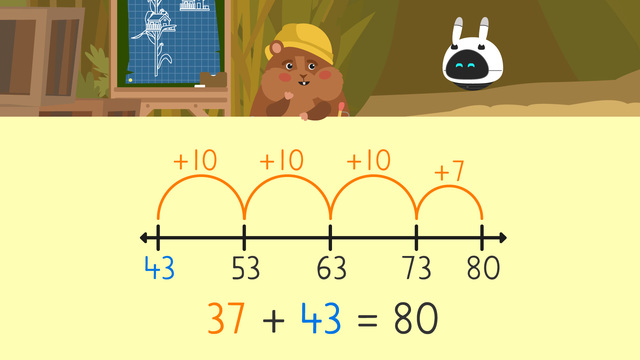# Make a Number Line to Add

Content Make a Number Line to AddRating

Give us a rating!
The authorsTeam Digital
Make a Number Line to Add
CCSS.MATH.CONTENT.2.OA.A.1:

## What do you learn about making a number line to add?

There are many tools and strategies to solve addition problems, one of which is using a number line! When using a number line to add...

• Start by drawing a number line.

• Next, put the larger addend on the left side under the first dash.

• Then, skip count forward the smaller addend.

• Finally, the number that you land on is your sum, or the answer to your equation!

## Make a Number Line to Add exercise

Would you like to apply the knowledge you’ve learned? You can review and practice it with the tasks for the video Make a Number Line to Add.
• ### What are the steps to make a number line to add?

Hints

What do we need to do before we put the larger addend on the left?

What do we need to do with the smaller addend in order to get our sum?

Solution

First, we draw a number line.

Second, we put the larger addend on the left. In this problem, 43.

Third, we skip count the smaller addend. In this problem +10 three times and then +7 (making 37).

Finally, we find our sum, which in this problem, is 80.

• ### Use the number line to find the sum.

Hints

Here you can see that 56 + 10 is 66.

Keep counting up to find your sum!

Finding your sum is the last step, so look for the last number on the number line to find your solution.

Solution

The answer is 88! We put 56, our larger addend, first and count up by 10s and then 2 in order to fill in our number line.

• ### Can you use a number line to find the sum?

Hints

Remember to start by counting by intervals of 10 to reach your final answer!

Be sure to identify which is the larger addend and start by putting that on the left.

Solution

22 + 34 = 56

• We put 34 to the left of our number line because it is the larger addend.
• Then we count up by 10 two times: 44, 54.
• We have 2 more seeds remaining, so we add those 2 to get our final answer, 56.
Mr. Squeaks has 56 seeds in total.
• ### Can you use a number line to find the sum?

Hints

Make sure that you put the largest addend on the left.

Remember that you need to skip count to find your solution. Use the number line above to help.

Solution
• First we find our largest addend 57 and put it on the left of our number line.
• Then we skip count by 10 three times and reach 87.
• We still need to add 5 more, which gives us our answer: 92 nails.
Imani has 92 nails.
• ### Solve the word problem.

Hints

If Mr. Squeaks has fewer cookies than Imani, that means that Imani has more cookies than Mr. Squeaks, so we need to add these numbers.

Solution

• ### Solve the word problem.

Hints

You can separate this problem into two separate number lines on your own if it makes it easier to solve.

Start with 25 and + 10 three times. You count by 10 three times because you're adding 2 tens from 20 and 1 ten from 12.

Solution
• First, we identify our largest addend, 25 bracelets.
• Then we skip count by 10 to add the 20 more bracelets Imani made. This leads us to 45 bracelets.
• We then skip count again by 10 to reach 55 bracelets.
• We need to add 2 more.